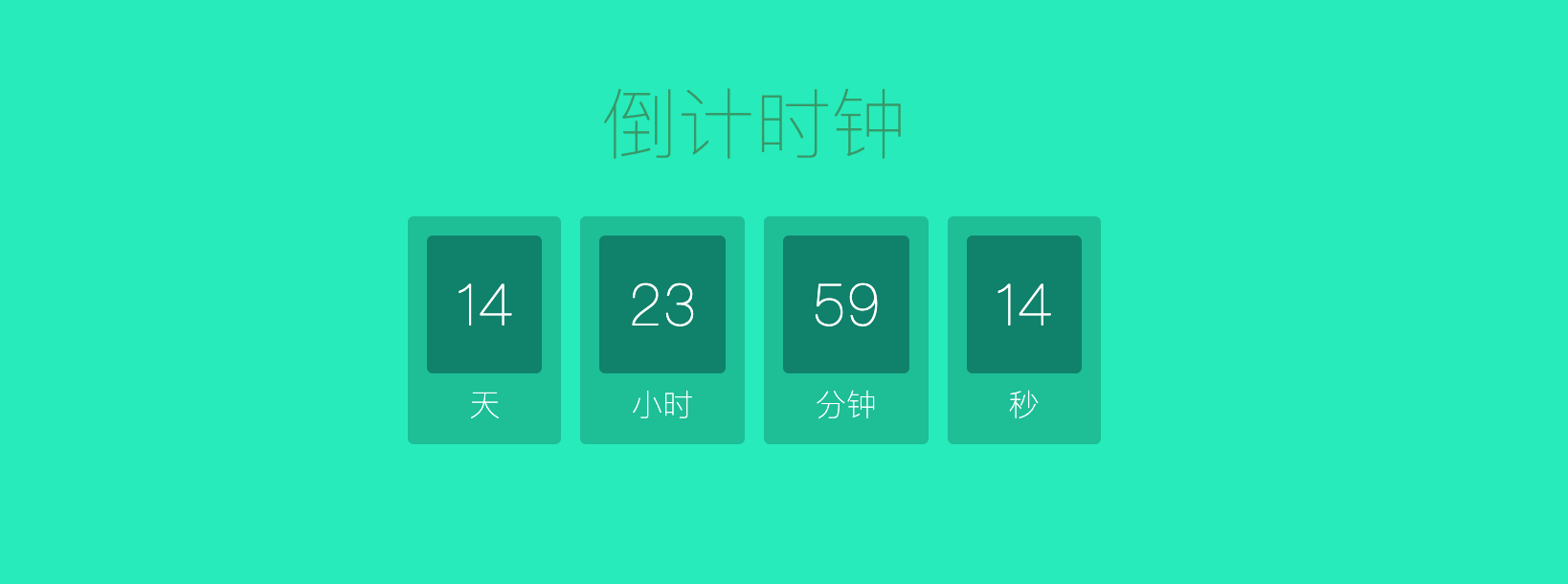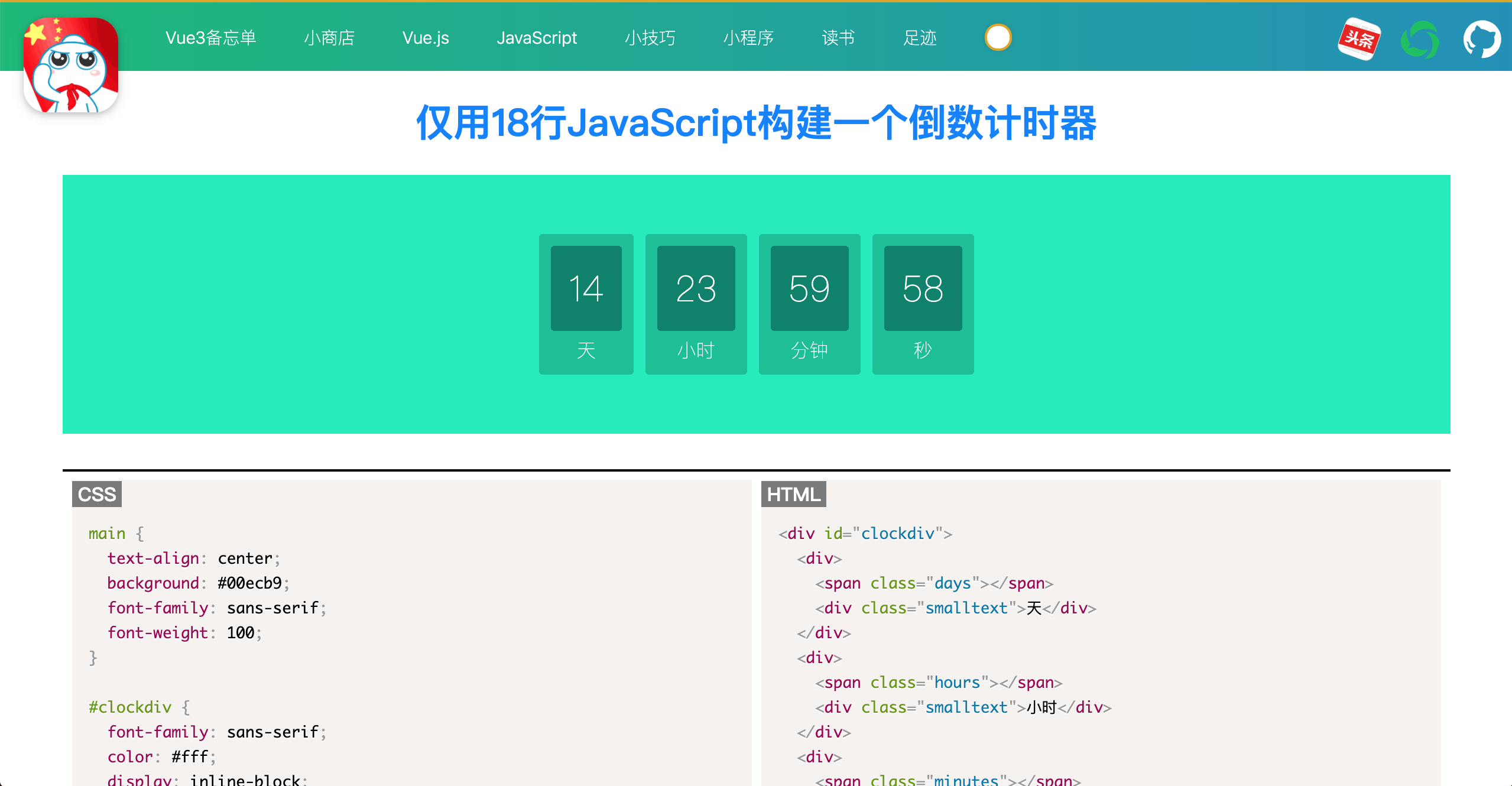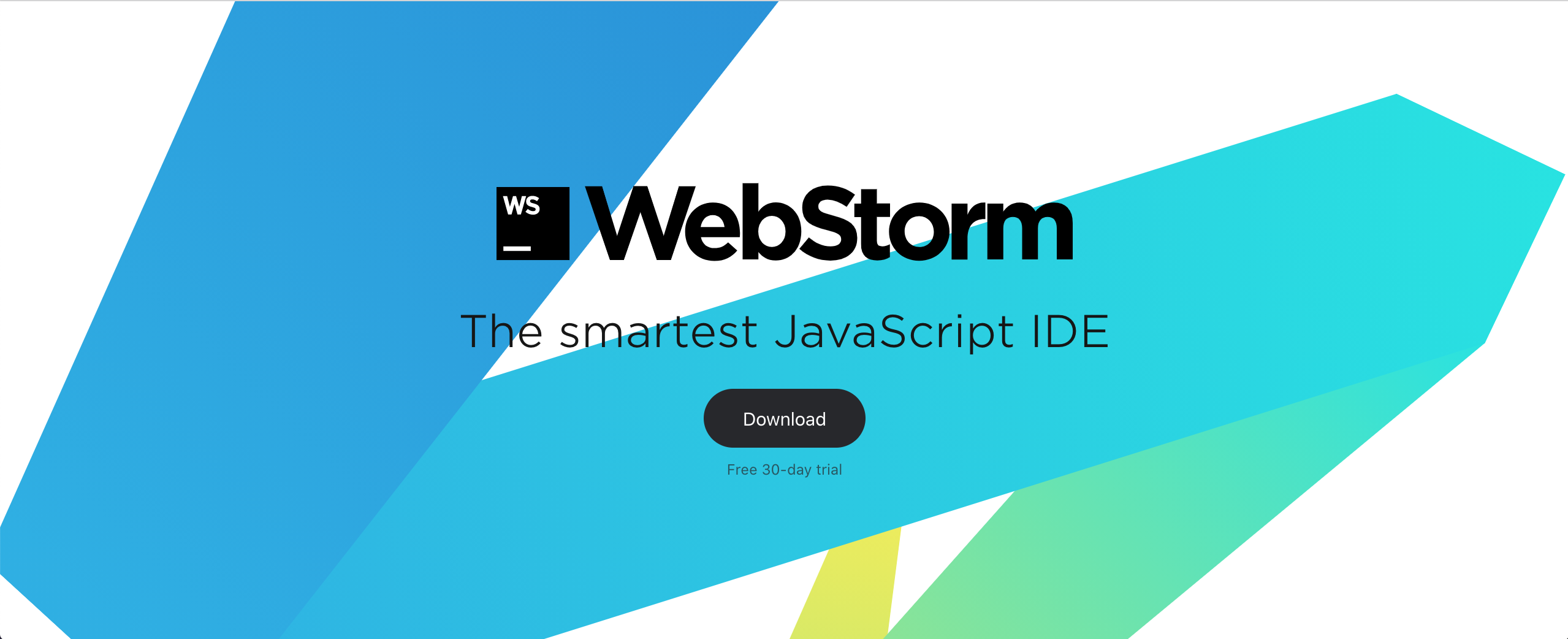# 实战|仅用18行JavaScript构建一个倒数计时器

• 你的代码将是轻量级的，因为它将具有零依赖性。
• 你的网站将表现得更好。你不需要加载外部脚本和样式表。
• 你将拥有更多的控制权。你将会建立一个完全按照你的意愿来表现的时钟。## 1.基本时钟：倒数到特定的日期或时间

• 设置有效的结束日期。
• 计算剩余时间。
• 将时间转换为可用格式。
• 将时钟数据输出为可重复使用的对象。
• 在页面上显示时钟，并在时钟为零时停止时钟。

## 2.设置有效的结束日期

ISO 8601格式：

``````const deadline = "2015-12-31";
``````

``````const deadline = "31/12/2015";
``````

``````const deadline = "December 31 2015";
``````

``````const deadline = "December 31 2015 23:59:59 GMT+0200";
``````

## 3.计算剩余时间

``````function getTimeRemaining(endtime) {
const total =
Date.parse(endtime) - Date.parse(new Date());
const seconds = Math.floor((total / 1000) % 60);
const minutes = Math.floor((total / 1000 / 60) % 60);
const hours = Math.floor((total / (1000 * 60 * 60)) % 24);
const days = Math.floor(total / (1000 * 60 * 60 * 24));

return {
total,
days,
hours,
minutes,
seconds,
};
}
``````

``````const total = Date.parse(endtime) - Date.parse(new Date());
``````

## 4.将时间转换为可用格式

``````const seconds = Math.floor((t / 1000) % 60);
``````

1. 将毫秒除以 1000 可转换为秒：`(t/1000)`
2. 将总秒数除以 60，然后取余数。你不需要所有的秒，只需要计算分钟数后剩下的那些：`(t/1000) % 60`
3. 四舍五入到最接近的整数。这是因为你需要完整的秒数，而不是几分之一秒：`Math.floor((t/1000)％60)`

## 5.将时钟数据输出为可重复使用的对象

``````return {
total,
days,
hours,
minutes,
seconds,
};
``````

``````getTimeRemaining(deadline).minutes;
``````

## 6.在页面上显示时钟，并在时钟为零时停止时钟

``````<div id="clockdiv"></div>
``````

``````function initializeClock(id, endtime) {
const clock = document.getElementById(id);
const timeinterval = setInterval(() => {
const t = getTimeRemaining(endtime);
clock.innerHTML =
"days: " +
t.days +
"<br>" +
"hours: " +
t.hours +
"<br>" +
"minutes: " +
t.minutes +
"<br>" +
"seconds: " +
t.seconds;
if (t.total <= 0) {
clearInterval(timeinterval);
}
}, 1000);
}
``````

• 计算剩余时间。
• 将剩余时间输出到我们的 div。
• 如果剩余时间为零停止计时。

``````initializeClock("clockdiv", deadline);
``````

## 7.准备展示你的时钟

• 消除初始延迟，使你的时钟立即显示。
• 让时钟脚本更有效率，这样它就不会连续重建整个时钟。
• 根据需要添加前导零。

### 7.1 消除初始延迟

``````const timeinterval = setInterval(() => { ... },1000);
``````

``````function updateClock() {
const t = getTimeRemaining(endtime);
clock.innerHTML =
"days: " +
t.days +
"<br>" +
"hours: " +
t.hours +
"<br>" +
"minutes: " +
t.minutes +
"<br>" +
"seconds: " +
t.seconds;
if (t.total <= 0) {
clearInterval(timeinterval);
}
}

updateClock(); // 首先运行一函数能以避免延迟
var timeinterval = setInterval(updateClock, 1000);
``````

### 7.2 避免不断重建时钟

``````<div id="clockdiv">
Days: <span class="days"></span><br />
Hours: <span class="hours"></span><br />
Minutes: <span class="minutes"></span><br />
Seconds: <span class="seconds"></span>
</div>
``````

``````const daysSpan = clock.querySelector(".days");
const minutesSpan = clock.querySelector(".minutes");
const secondsSpan = clock.querySelector(".seconds");
``````

``````function updateClock(){
const t = getTimeRemaining(endtime);

daysSpan.innerHTML = t.days;
minutesSpan.innerHTML = t.minutes;
secondsSpan.innerHTML = t.seconds;

...
}
``````

### 7.3 添加前导 0

``````secondsSpan.innerHTML = t.seconds;
``````

``````secondsSpan.innerHTML = ("0" + t.seconds).slice(-2);
``````

## 8.更进一步

### 8.1 自动调节时钟

``````clock.style.display = "block";
``````

``````const schedule = [
["Jul 25 2015", "Sept 20 2015"],
["Sept 21 2015", "Jul 25 2016"],
["Jul 25 2016", "Jul 25 2030"],
];
``````

`Schedule` 数组中的每个元素代表一个开始日期和一个结束日期。如上所述，它可以包含时间和时区，但我在这里使用了普通的日期，以保持代码的可读性。

``````// 遍历schedule中的每个元素
schedule.forEach(([startDate, endDate]) => {
// 以毫秒为单位放置日期以便于比较
const startMs = Date.parse(startDate);
const endMs = Date.parse(endDate);
const currentMs = Date.parse(new Date());

// 如果当前日期在开始日期和结束日期之间，则显示时钟
if (endMs > currentMs && currentMs >= startMs) {
initializeClock("clockdiv", endDate);
}
});
``````

### 8.2 从用户到达起将计时器设置为 10 分钟

``````const timeInMinutes = 10;
const currentTime = Date.parse(new Date());
currentTime + timeInMinutes * 60 * 1000
);
``````

### 8.3 跨页面保持时钟进度

``````let deadline;

/(^|;)myClock=([^;]+)/
);
} else {
// 否则，请设置从现在开始10分钟的截止日期，

// 创建从现在开始10分钟的截止日期
const timeInMinutes = 10;
const currentTime = Date.parse(new Date());
currentTime + timeInMinutes * 60 * 1000
);

"myClock=" +
"; path=/; domain=.yourdomain.com";
}
``````

## 9.有关客户端时间的重要警告

JavaScript 日期和时间是从用户的计算机上获取的，这意味着用户可以通过更改计算机上的时间来影响 JavaScript 时钟。在大多数情况下，这并不重要，但在一些超级敏感的情况下，就需要从服务器上获取时间。可以使用一些 Node.js 或 Ajax 来完成，这两者都超出了本教程的范围。

## 11.代码上一篇如何在WebStorm中获得对数据库工具和SQL的支持

2020-10-26译|通过Node和Redis进行API速率限制

2020-09-16
目录